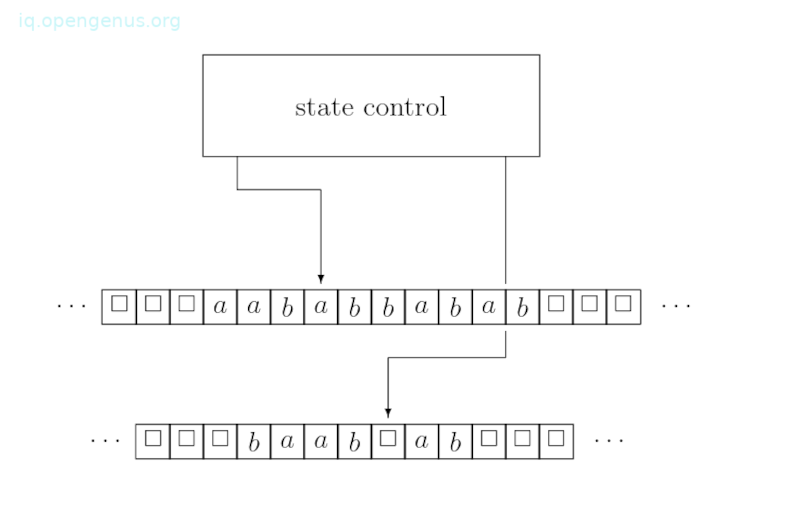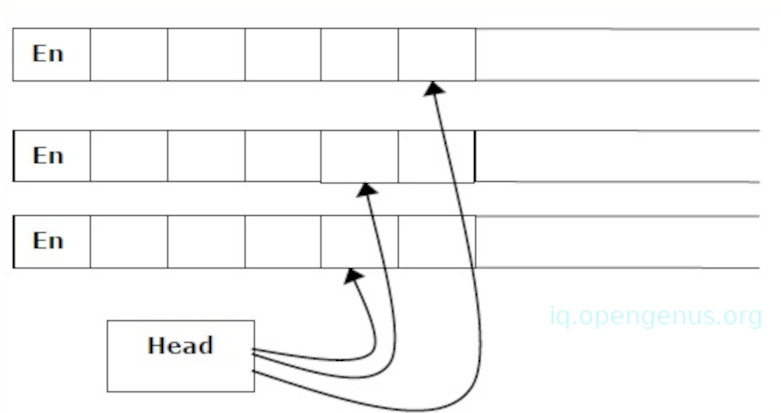×

Search anything:

# Turing Machines and Church-Turing Thesis in Theory of Computation

#### Theory of ComputationFREE BOOK -> Problems for the day before your Coding Interview (on Amazon)

A turing machine is a mathematical model of a computation that defines an abstract machine. In this article, we learn about Turing machines, how they are defined formally and informally, and the Church-Turing thesis.

1. Introduction.
2. Formal and informal definitions.
3. Multi-tape turing machines.
4. Formal definition of multi-tape turing machines.
5. The Church-Turing thesis.
6. Summary.
7. References.

## Introduction.

In the prerequisite articles, we learned about regular and context-free languages. We learned of computational devices that accept or generate these languages and their limitations when it comes to languages such as; A = {${\mathrm{a}}^{\mathrm{m}}$${\mathrm{b}}^{\mathrm{n}}$${\mathrm{c}}^{\mathrm{mn}}$ : m ≥ 0, n ≥ 0}.

A turing machine models a real computer. These machines accept all context-free grammar as well as languages such as A.

In this article, we will try to prove that "every problem solvable by a real computer is solvable by a turing machine" - this is the church turing thesis.

## Formal and informal definitions.

A turing machine will consist of the following;

1. First we have k tapes, each tape divided into cells. This tape is infinite on both sides left and right. Each cell in the tape stores a symbol that belongs to a finite set T referred to as the tape alphabet. This alphabet has a blank symbol ⋄ If a cell has this symbol, it is considered empty.

Let's look at an example of a turing machine where k is 2;1. Each tape has a tape that can be moved along the other tape. It only moves a single cell per move. It reads the cell it is currently positioned at and replaces the symbol in the cell with another symbol.

2. There is also a state control that can be any of a finite number of states. A finite set of states is denoted by Q, this set has three special states namely, start, accept, and reject.

During a single step of computation the turing machine does the following;

1. At the beginning it is in a state r of Q and each k tape head is on a specific cell.
2. Depending on the current state r and k symbols;
• The machine switches to state r' of Q
• Each tape head writes a symbol T in the cell it is currently scanning.
• Each tape head can move one cell to the left, right, or stay where it currently is.

#### A formal definition.

A deterministic turing machine is 7-tuple consisting of M = (Σ, Γ, Q, δ, q, ${\mathrm{q}}_{\mathrm{accept}}$, ${\mathrm{q}}_{\mathrm{reject}}$).
Where;

1. Σ is a finite set referred to as an input alphabet.
2. Γ is a finite set referred to as a tape alphabet, it has the blank symbol ⋄ and Σ ⊆ Γ.
3. Q is a finite set whose elements are referred to as states.
4. q is an element of Q referred to as the start state.
5. ${\mathrm{q}}_{\mathrm{accept}}$ is an element of Q, it is referred to as the accept state.
6. ${\mathrm{q}}_{\mathrm{reject}}$ is also an element of Q, it is referred to as the reject state.
7. δ is referred to as a transition function.
δ:Q × ${\mathrm{\Gamma }}^{\mathrm{k}}$→ Q × ${\mathrm{\Gamma }}^{\mathrm{k}}$ × ${\mathrm{\left\{L, R, N\right\}}}^{\mathrm{k}}$.
This function is a program of the turing machine, it tells the machine what it can do in one computation step.

## Multi-tape turing machines.

These machines have multiple tapes each accessed with a separate head that moves independently despite the other heads.

In the beginning, the input is at tape 1 while others remain blank. In the next step, the machine reads consecutive symbols under its head and prints a symbol on each tape then moves its head.Although it is easier to obtain a two-tape machine compared to a single-tape turing machine, this doesn't mean that a two-tape machine is more powerful.

## A formal definition of multi-tape turing machines.

This machine is 6-tuple (Q, X, B, δ, q0, F) where;

1. Q is a finite set of states.
2. X is the tape alphabet.
3. B is the blank symbol.
4. δ is a relation between states and symbols where;
δ: Q × Xk → Q × (X × {Leftshift, Rightshift, Noshift })k where there is k number of tapes.
5. q0 is the initial state.
6. F is the set of final states.

Note that for every multi-tape turing machine, there is an equivalent single-tape turing machine.

## The Church-Turing thesis.

An algorithm is a list of steps describing how to solve a problem with a computer and therefore any computational process that can be specified by a program is considered an algorithm.

Similarly, a turing machine specifies a computational process and therefore we consider it as an algorithm.

Now, we ask. Is it possible to give a mathematical definition of an algorithm?
Having stated that every program be it a java program or C program represents an algorithm and that every turing machine represents an algorithm. We ask ourselves, are these two statements regarding an algorithm equivalent?

The answer is yes, it also implies that many different notions of computational processes are equivalent.

For example, the following are computational models.

• One-tape turing machines.
• Non-deterministic turing machines.
• k-tape turing machines where k >= 1.
• Java, C++ programs.

Any of the models can be converted to any other model.

The Church-Turing thesis: It states that every computational process that is intuitively considered an algorithm can be converted to a turing machine.
In other terms, we define an algorithm to be a turing machine.

## Summary.

A turing machine is a mathematical model of a computation that defines an abstract machine. Despite its simplicity, given any algorithm, this machine is capable of implementing the algorithm's logic.

The Church-Turing thesis states that every computational process that is said to be an algorithm can be implemented by a turing machine.

#### Erick Lumunge

Erick is a passionate programmer with a computer science background who loves to learn about and use code to impact lives positively.

Turing Machines and Church-Turing Thesis in Theory of Computation